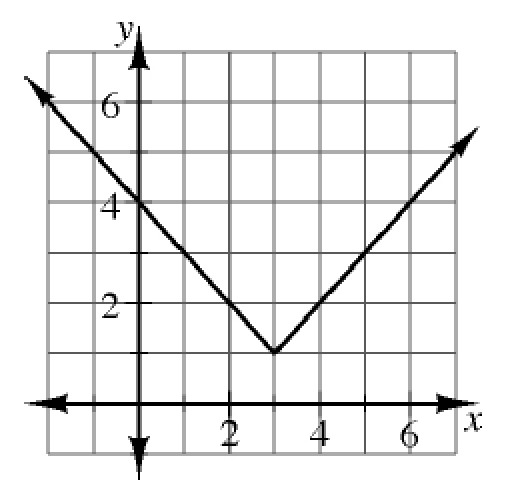### Home > INT3 > Chapter 3 > Lesson 3.1.3 > Problem3-50

3-50.

Examine the graph of $f\left(x\right)=\left|x-3\right|+1$ at right. Explain how you can use the graph to determine the values below.

1. $f\left(3\right)$

What $y$-value corresponds with $x=3$?

$1$

1. $f\left(0\right)$

$4$

1. $f\left(4\right)$

Refer to parts (a) and (b).

1. $f\left(-1\right)$

Refer to parts (a) and (b).